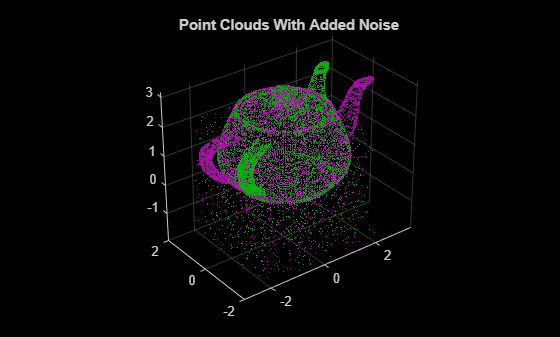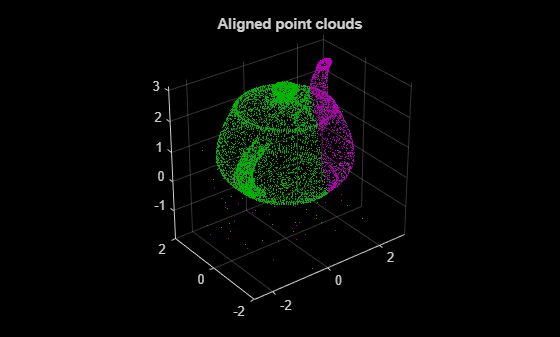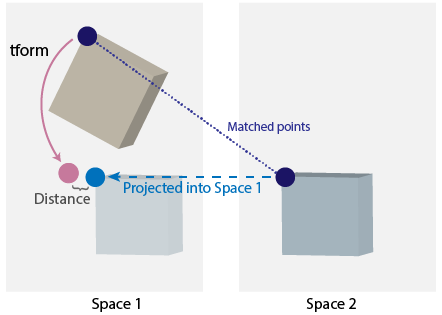Main Content

# estimateGeometricTransform3D

Estimate 3-D geometric transformation from matching point pairs

## Syntax

``tform = estimateGeometricTransform3D(matchedPoints1,matchedPoints2,transformType)``
``````[tform,inlierIndex] = estimateGeometricTransform3D(___)``````
``[tform,inlierIndex,status] = estimateGeometricTransform3D(___)``
``[___] = estimateGeometricTransform3D(___, Name,Value)``

## Description

example

````tform = estimateGeometricTransform3D(matchedPoints1,matchedPoints2,transformType)` estimates a 3-D geometric transformation between two sets of 3-D points by mapping the inliers in the matched points from one set of 3-D points `matchedPoints1` to the inliers in the matched points from the other set of 3-D points `matchedPoints2`.```
``````[tform,inlierIndex] = estimateGeometricTransform3D(___)``` additionally returns a vector specifying each matched point pair as either an inlier or an outlier using the input arguments from the previous syntax.```
````[tform,inlierIndex,status] = estimateGeometricTransform3D(___)` additionally returns a status code indicating whether or not the function could estimate a transformation and, if not, why it failed. If you do not specify the `status` output, the function instead returns an error for conditions that cannot produce results.```
````[___] = estimateGeometricTransform3D(___, Name,Value)` specifies additional options using one or more name-value pair arguments in addition to any combination of arguments from previous syntaxes. For example, `'Confidence',99` sets the confidence value for finding the maximum number of inliers to `99`.```

## Examples

collapse all

Load a point cloud file into the workspace.

`ptCloud1 = pcread('teapot.ply')`
```ptCloud1 = pointCloud with properties: Location: [41472×3 single] Count: 41472 XLimits: [-3 3.4340] YLimits: [-2 2] ZLimits: [0 3.1500] Color: [] Normal: [] Intensity: [] ```
`ptCloud1 = pcdownsample(ptCloud1,'random',0.25); `

Create a rigid 3-D transformation object with a 30-degree rotation.

```theta = 30; % degrees rot = [cosd(theta) sind(theta) 0; ... -sind(theta) cosd(theta) 0; ... 0 0 1]; trans = [0 0 0]; tform = rigid3d(rot,trans);```

Transform the point cloud using the transformation object.

`ptCloud2 = pctransform(ptCloud1,tform);`

To introduce noise, add random points to both point clouds.

```noise1 = rescale(rand(1000,3),-2,2); ptCloud1 = pointCloud([ptCloud1.Location;noise1]); noise2 = rescale(rand(1000,3),-2,2); ptCloud2 = pointCloud([ptCloud2.Location;noise2]);```

Visualize the noisy point clouds.

```figure pcshowpair(ptCloud1,ptCloud2) title('Point Clouds With Added Noise')```Extract matched points from the point clouds.

```matchedPoints1 = ptCloud1.Location; matchedPoints2 = ptCloud2.Location;```

Estimate the rigid transformation between the point clouds.

```[tformEst,inlierIndex] = estimateGeometricTransform3D(matchedPoints1, ... matchedPoints2,'rigid'); ```

Extract the inlier points.

```inliersPtCloud1 = transformPointsForward(tformEst,matchedPoints1(inlierIndex,:)); inliersPtCloud2 = matchedPoints2(inlierIndex,:);```

Visualize the inliers of the aligned point clouds.

```figure firstPtCloud = pointCloud(inliersPtCloud1); secondPtCloud = pointCloud(inliersPtCloud2); pcshowpair(firstPtCloud,secondPtCloud) title('Aligned point clouds')```## Input Arguments

collapse all

First set of matched 3-D points, specified as an M-by-3 matrix in which each row is a set of (x,y,z) coordinates and M is the number of matched points.

Second set of matched 3-D points, specified as an M-by-3 matrix in which each row is a set of (x,y,z) coordinates and M is the number of matched points.

Transformation type, specified as `'rigid'` or `'similarity'`. Each transform type requires a minimum number of matched pairs of points to estimate a transformation. You can generally improve the accuracy of a transformation by using a larger number of matched pairs of points. This table shows the type of object associated with each transformation type and the minimum number of matched pairs of points the transformation requires.

`transformType``tform` ObjectMinimum Number of Matched Pairs of Points
`'rigid'``rigid3d`3
`'similarity'``affine3d`3

Data Types: `string`

### Name-Value Pair Arguments

Specify optional comma-separated pairs of `Name,Value` arguments. `Name` is the argument name and `Value` is the corresponding value. `Name` must appear inside quotes. You can specify several name and value pair arguments in any order as `Name1,Value1,...,NameN,ValueN`.

Example: `'Confidence'`,`99` sets the confidence value for finding the maximum number of inliers to `99`.

Maximum number of random trials, specified as the comma-separated pair consisting of `'MaxNumTrials'` and a positive integer. This value specifies the number of randomized attempts the function makes to find matching point pairs. Specifying a higher value causes the function to perform additional computations, which increases the likelihood of finding inliers.

Data Types: `single` | `double` | `int8` | `int16` | `int32` | `int64` | `uint8` | `uint16` | `uint32` | `uint64`

Confidence of finding the maximum number of inliers, specified as the comma-separated pair consisting of `'Confidence'` and a positive numeric scalar in the range (0, 100). Increasing this value causes the function to perform additional computations, which increases the likelihood of finding a greater number of inliers.

Data Types: `single` | `double` | `int8` | `int16` | `int32` | `int64` | `uint8` | `uint16` | `uint32` | `uint64`

Maximum distance from a point to the projection of the corresponding point, specified as the comma-separated pair consisting of `'MaxDistance'` and a positive numeric scalar. `'MaxDistance'` specifies the maximum distance, in pixels, that a point can differ from the projected location of its corresponding point to be considered an inlier. The corresponding projection is based on the estimated transform.

The function checks for a transformation from `matchedPoints1` to `matchedPoints2`, and then calculates the distance between the matched points in each pair after applying the transformation. If the distance between the matched points in a pair is greater than the `'MaxDistance'` value, then the pair is considered an outlier for that transformation. If the distance is less than `'MaxDistance'`, then the pair is considered an inlier.Data Types: `single` | `double` | `int8` | `int16` | `int32` | `int64` | `uint8` | `uint16` | `uint32` | `uint64`

## Output Arguments

collapse all

Geometric transformation, returned as an `affine3d` or a `rigid3d` object.

The returned geometric transformation matrix maps the inliers in `matchedPoints1` to the inliers in `matchedPoints2`. The function returns an object specific to the transformation type specified by the `transformType` input argument.

`transformType``tform`
`'rigid'``rigid3d`
`'similarity'``affine3d`

Status code, returned as `0`, `1`, or `2`. The status code indicates whether or not the function could estimate the transformation and, if not, why it failed.

ValueDescription
`0`No error
`1``matchedPoints1` and `matchedPoints2` inputs do not contain enough points
`2`Not enough inliers found

If you do not specify the `status` code output, the function returns an error if it cannot produce results.

Data Types: `int32`

Inliers, returned as an M-by-1 logical vector of M point pairs. Each element contains either a logical true, `1`, to indicate the point pair is an inlier, or a logical false, `0`, to indicate the point pair is an outlier.

## Algorithms

The function excludes outliers using the M-estimator sample consensus (MSAC) algorithm. The MSAC algorithm is a variant of the random sample consensus (RANSAC) algorithm. Results may not be identical between runs due to the randomized nature of the MSAC algorithm.

 Hartley, Richard, and Andrew Zisserman. Multiple View Geometry in Computer Vision. 2nd ed. Cambridge, UK ; New York: Cambridge University Press, 2003.

 Torr, P.H.S., and A. Zisserman. “MLESAC: A New Robust Estimator with Application to Estimating Image Geometry.” Computer Vision and Image Understanding 78, no. 1 (April 2000): 138–56. https://doi.org/10.1006/cviu.1999.0832.

Download ebook